# Factoring Primes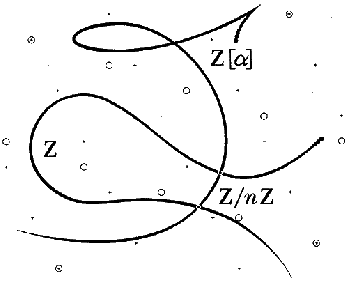A diagram from [LL93].

 The obvious mathematical breakthrough would be development of an easy way to factor large prime numbers.'' -Bill Gates, The Road Ahead, pg. 265Let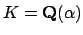be a number field, and let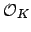be the ring of integers of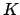. To employ our geometric intuition, as the Lenstras did on the cover of [LL93], it is helpful to viewas a one-dimensional scheme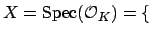all prime ideals of $O_K$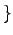over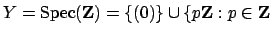is prime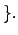There is a natural map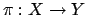that sends a prime ideal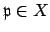to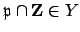. For much more on this point of view, see [EH00, Ch. 2].

Ideals were originally introduced by Kummer because, as we proved last Tuesday, in rings of integers of number fields ideals factor uniquely as products of primes ideals, which is something that is not true for general algebraic integers. (The failure of unique factorization for algebraic integers was used by Liouville to destroy Lamé's purported 1847 proof'' of Fermat's Last Theorem.)

If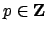is a prime number, then the ideal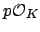offactors uniquely as a product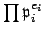, where the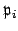are maximal ideals of. We may imagine the decomposition ofinto prime ideals geometrically as the fiber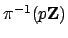(with multiplicities).

How can we computein practice?

Example 8.1.1   The following session shows the commands needed to compute the factorization ofin forthe number field defined by a root of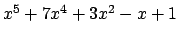.
   > R<x> := PolynomialRing(RationalField());
> K<a> := NumberField(x^5 + 7*x^4 + 3*x^2 - x + 1);
> OK := MaximalOrder(K);
> I := 2*OK;
> Factorization(I);
[
<Principal Prime Ideal of OK
Generator:
[2, 0, 0, 0, 0], 1>
]
> J := 5*OK;
> Factorization(J);
[
<Prime Ideal of OK
Two element generators:
[5, 0, 0, 0, 0]
[2, 1, 0, 0, 0], 1>,
<Prime Ideal of OK
Two element generators:
[5, 0, 0, 0, 0]
[3, 1, 0, 0, 0], 2>,
<Prime Ideal of OK
Two element generators:
[5, 0, 0, 0, 0]
[2, 4, 1, 0, 0], 1>
]
> [K!OK.i : i in [1..5]];
[ 1, a, a^2, a^3, a^4 ]

Thus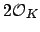is already a prime ideal, and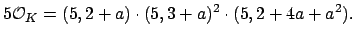Notice that in this example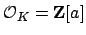. (Warning: There are examples ofsuch that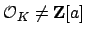for any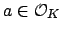, as Example 8.1.6 below illustrates.) Whenit is very easy to factor, as we will see below. The following factorization gives a hint as to why: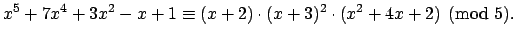The exponent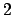of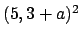in the factorization of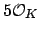above suggests ramification'', in the sense that the cover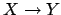has less points (counting their size'', i.e., their residue class degree) in its fiber over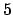than it has generically. Here's a suggestive picture: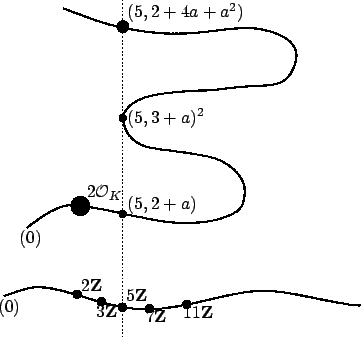Diagram of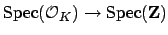Subsections
William Stein 2004-05-06